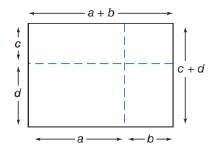Chapter 8.1, Problem 45EElementary Geometry For College St...

7th Edition
Alexander + 2 others
ISBN: 9781337614085

Solutions

Chapter
SectionElementary Geometry For College St...

7th Edition
Alexander + 2 others
ISBN: 9781337614085
Textbook Problem

The algebra method of FOIL multiplication is illustrated geometrically in the drawing. Use the drawing with rectangular regions to complete the following rule: ( a + b ) ( c + d ) = _ _ _ _ _ _ _ _ _ _ _ _ _ _ _ _ _ _ _ _ _ _ _ _To determine

To complete:

(a+b)(c+d)=_ by the algebra method of FOIL multiplication is illustrated geometrically in the drawing.

Explanation

The area of the rectangle A=lw unit2.

Where l is the length of the rectangle, w is the width of the rectangle.

Multiplication of the terms is followed by the FOIL method,

Calculation:

Given,

By the drawing which is a rectangle whose length l=(a+b) and width w=(c+d)

The area of the rectangle =lw unit2.

(i.e.) A=(a+b)(c+d)

By the FOIL method,

=ac+

Still sussing out bartleby?

Check out a sample textbook solution.

See a sample solution

The Solution to Your Study Problems

Bartleby provides explanations to thousands of textbook problems written by our experts, many with advanced degrees!

Get Started

(t+6)(60)(60t+180)(t+6)2

Applied Calculus for the Managerial, Life, and Social Sciences: A Brief Approach

Find the limit. a. limx15 b. limx6x c. limx5x2 d. limx8x3

Calculus: An Applied Approach (MindTap Course List)

Differentiate the function. h(t)=t44et

Single Variable Calculus: Early Transcendentals

The graph of x = 2 + 3t, y = 4 − t is a: circle ellipse line parabola

Study Guide for Stewart's Single Variable Calculus: Early Transcendentals, 8th